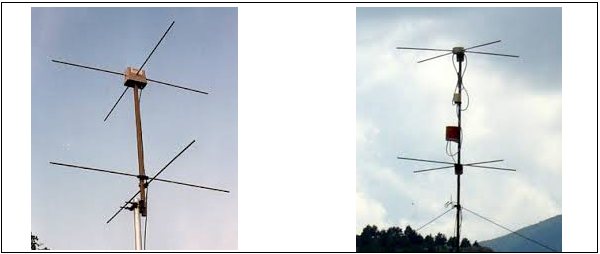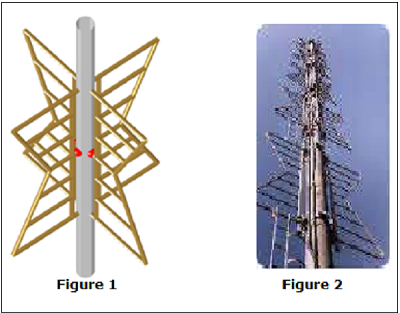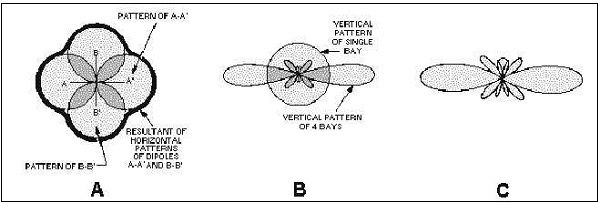# Antenna Theory - Turnstile Antenna

The Turnstile antenna is another type of array antenna. The shape of this array symbolizes the turnstile, which is used at the entrances of few places. This antenna has a wide variety of military applications.

### Frequency range

The frequency range in which the turnstile antennas operate is around 30 MHz to 3GHz which belong to the VHF and UHF bands.

## Construction & Working of Turnstile Antenna

Two identical half-wave dipoles are placed at right angles to each other and are fed inphase. These dipoles are excited 90° out of phase with each other. Turnstile array can also be termed as crossed dipoles array.The above images illustrate turnstile antennas.

To provide high directivity, several turnstiles may be stacked along a vertical axis, and are phased as shown in the figure given above. The polarization of these turnstile antennas depend upon their mode of operation.

The pair of such dipoles frequently stacked, is known as BAY. In the figures shown above, two bays are spaced half wavelength (λ/2) apart and the corresponding elements are fed in phase. The radiation produce by the combination of bays results in better directivity.

## Modes of Operation

The following are the modes of operation of a Turnstile antenna.

Normal mode

In Normal mode of operation, the antenna radiates horizontally polarized waves which are perpendicular to its axis.

Axial mode

In Axial mode of operation, the antenna radiates circularly polarized waves along its axis i.e. parallel to its axis.

For circular polarization, the transmitter radiating with right-circular polarization should have a receiver with same right-circular polarization and vice versa. If it is left-circular polarized one, unlike the transmitter, there will be a severe loss of gain.

## Super Turnstile Antenna

For a turnstile antenna, the radiation power is 3dB below the maximum radiation of a halfwave dipole radiating the same power. Therefore, to overcome this disadvantage, the Super-turnstile antenna is built.

The simple dipole elements in turnstile are replaced by four flat sheets in Super-turnstile. The design of Super-turnstile array is such that 1 to 8 bays can be constructed on a single mast. The other name for Super-turnstile antenna is the Batwing Antenna.The above images show super-turnstile antenna. Figure 1 shows the arrangement of superturnstile array with the red dots being the feed points. Figure 2 shows the stacked turnstile array used in satellite communications.

The radiation pattern will be similar to the radiation pattern of two super imposed dipoles. Though it is close to omni-directional pattern, it leaves a cloveleaf shaped pattern.The above figure shows the radiational pattern of a turnstile array. The typical figure-ofeight patterns were combined to produce a nearly circular pattern.

• Figure A shows the individual patterns being combined.

• Figure B shows the vertical pattern of single bay and also the combined pattern of four bays.

• Figure C shows the resultant combined pattern of four bays showing better directivity.

The following are the advantages of Turnstile antennas −

• High-gain is achieved by stacking

• Super-turnstile produces high-gain output

• Better directivity is achieved

The following is the disadvantage of Turnstile antennas −

• The radiation power is 3dB below the maximum radiation of a half wave dipole radiating the same power.

### Applications

The following are the applications of Turnstile antennas −

• Used for VHF communications

• Used for FM and TV broadcasting

• Used in military communications

• Used in satellite communications